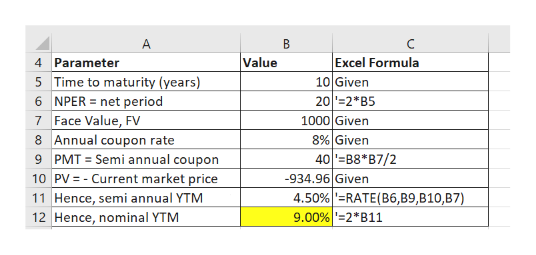A bond has an annual 8 percent coupon rate, a maturity of 10 years, a face value of \$1000 and makes semi-annual payments.  If the price is \$934.96, what is the annual nominal yield to maturity?

Question

A bond has an annual 8 percent coupon rate, a maturity of 10 years, a face value of \$1000 and makes semi-annual payments.  If the price is \$934.96, what is the annual nominal yield to maturity?

Step 1

Please note that coupons are being paid semi annually. Hence, the period here is half year. All the parameters need to be expressed as per period i.e. per half year.

Step 2

We can calculate the yield to maturity (YTM) using the RATE function of excel.

Please see the white board. Yellow colo...help_outlineImage TranscriptioncloseExcel Formula Value 4 Parameter 5 Time to maturity (years) 6 NPER net period 10 Given 20 2 B5 7 Face Value, FV 8 Annual coupon rate 9 PMT Semi annual coupon 10 PV-Current market price 11 Hence, semi annual YTM 12 Hence, nominal YTM 1000 Given 8% Given 40B8* B7/2 934.96 Given 4.50% =RATE(B6,B9,B10,B7) 9.00% 2B11 fullscreen

Want to see the full answer?

See Solution

Want to see this answer and more?

Our solutions are written by experts, many with advanced degrees, and available 24/7

See Solution
Tagged in